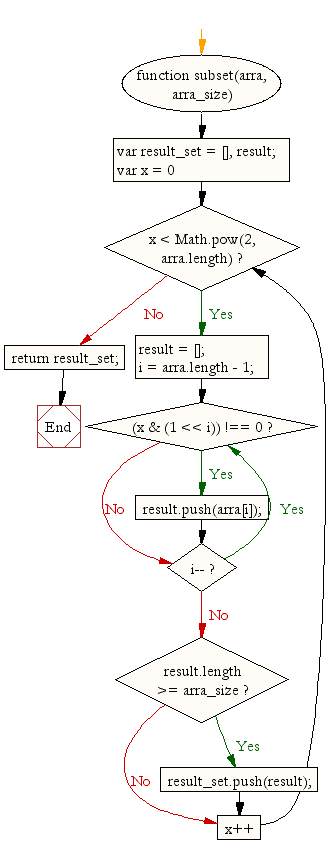# JavaScript: Get all possible subset with a fixed length combinations in an array

## JavaScript Function: Exercise-21 with Solution

Write a JavaScript function to get all possible subset with a fixed length (for example 2) combinations in an array.

Sample array : [1, 2, 3] and subset length is 2

Expected output : [[2, 1], [3, 1], [3, 2], [3, 2, 1]]

Sample Solution: -

HTML Code:

``````<!DOCTYPE html>
<html>
<meta charset="utf-8">
<title>JavaScript array subset</title>
<body>

</body>
</html>
```
```

JavaScript Code:

``````function subset(arra, arra_size)
{
var result_set = [],
result;

for(var x = 0; x < Math.pow(2, arra.length); x++)
{
result = [];
i = arra.length - 1;
do
{
if( (x & (1 << i)) !== 0)
{
result.push(arra[i]);
}
}  while(i--);

if( result.length >= arra_size)
{
result_set.push(result);
}
}

return result_set;
}

console.log(subset([1, 2, 3], 2));
```
```

Sample Output:

```[[2,1],[3,1],[3,2],[3,2,1]]
```

Flowchart:Live Demo:

See the Pen JavaScript - Get all possible subset with a fixed length combinations in an array-function-ex- 21 by w3resource (@w3resource) on CodePen.

Improve this sample solution and post your code through Disqus

What is the difficulty level of this exercise?

﻿

## JavaScript: Tips of the Day

Errors

```const name = 'Owen';

console.log(name());
```

The variable name holds the value of a string, which is not a function, thus cannot invoke.
TypeErrors get thrown when a value is not of the expected type. JavaScript expected name to be a function since we're trying to invoke it. It was a string however, so a TypeError gets thrown: name is not a function!
SyntaxErrors get thrown when you've written something that isn't valid JavaScript, for example when you've written the word return as retrun. ReferenceErrors get thrown when JavaScript isn't able to find a reference to a value that you're trying to access.

Ref: https://bit.ly/3jFRBje